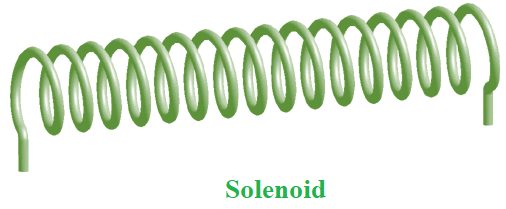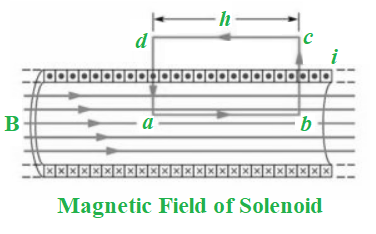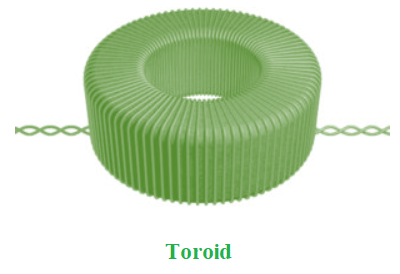# Magnetic Field Due to Solenoid and Toroid

A charge is surrounded by an electric field when it is sufficiently sluggish and sits idle. This would make sense to you because it is an electric charge. However, when that charge becomes excited and starts racing about, it generates a magnetic field. Doesn’t this strike you as strange? You aren’t alone, believe me! Both fields are part of the same force of nature, as physicists discovered later: electromagnetism! The notion of electromagnetism is used by a solenoid.

### Solenoid

The solenoid is a wire coil that works as an electromagnet when electricity flows through it. Electromagnetic solenoids are used in a variety of applications across the world.

It’s nearly impossible to swing a bat without striking a solenoid. Solenoids are found in both speakers and microphones. In reality, a speaker and a microphone are essentially the same things when seen from the opposite perspective. Solenoids are used in a variety of solenoid engines because the coils may be reinforced by adding an iron core. Solenoids, like bar magnets, are made up of a large coil of wire wrapped in many turns that generates a homogeneous magnetic field when electricity is delivered through it.### Types of Solenoids

Solenoids are available in a variety of designs, materials, and functions. However, they all operate on the same electrical principles:

1. AC laminated solenoid – What makes an AC solenoid renowned is the amount of force it can generate in its initial stroke. They emit a crisp buzz while in operation and are available in a variety of combinations and ranges. The stroke of an AC solenoid can be longer than that of a DC solenoid.
2. DC C-frame solenoid – A DC C-frame solenoid has merely a frame enclosing the coil in the shape of the letter C. They are most commonly used in a DC arrangement, although they may also be utilized in an AC power system.
3. DC D-frame solenoid – The coil is covered by two sections of frames in the DS D-frame solenoid. They’re frequently utilized in industrial settings. These solenoids may also be made to work with AC power.
4. Linear solenoid – These solenoids are used for metering activities and can apply a pulling or pushing force to mechanical equipment. These are the most popular solenoids, and they’re utilized in motor vehicle starters. These solenoids move linearly when an electrical force is applied to them, bringing two contacts together. This lets electricity from the battery flow into various sections of the vehicle, allowing it to start.
5. Rotary solenoid – This solenoid is utilized for applications that need a simple and automated control procedure. Among all varieties of solenoids, it is the most durable. They were originally intended for defense mechanisms, but they may today be found in a variety of automated industrial operations such as shutters and lasers.

### Amperian Loop to Determine the Magnetic Field

Consider an amperian loop and integrate along each side with sides abcd. The field in bc and da is perpendicular to dl. The field is zero along cd. The field component is 0 for transverse sections bc and ad. As a result, these two parts have no value. Let B be the field along ab. As a result, L = h is the appropriate length of the Amperian loop. The total number of turns is nh if n is the number of turns per unit length.Ie = I (n h) is the enclosed current where I is the current in the solenoid.

B L = μ0 Ie

B h = μ0 I (n h)

B = μ0 n I

The right-hand rule determines the field’s orientation. A solenoid is a device that is often used to create a consistent magnetic field.

### A long solenoid

The term “long solenoid” refers to a solenoid with a longer length than the radius. It is made out of a long wire coiled in the shape of a helix with closely spaced neighbouring turns. As a result, each turn may be thought of as a circular loop. The net magnetic field is the vector sum of all the turns’ fields. The turns are isolated from one other by using enamelled wires for winding.

The magnetic field within a long solenoid is,

B = μ0 n I

where, n denotes the number of turns per unit length and I denote the current flowing through the solenoid.

The magnetic field at one end of the long solenoid is as follows:

B = (μ0 n I)/2

Properties of a Solenoid

1. The magnetic fields of a solenoid are determined by the density of coils, the number of turns, and the current flowing through it.
2. Because they can transform electric current into mechanical motion, solenoids are frequently employed in switches.
3. Outside of the centre, the magnetic field lines are farther apart and the fields are weaker.
4. The long solenoid’s homogeneous magnetic field is located in the middle.
5. Even a tiny Solenoid may exert a few Newtons of force.
6. The force with which a ferromagnetic rod placed near a solenoid is drawn towards it may be estimated by measuring the change in magnetic field energy when the rod is introduced inside the solenoid:

Force on rod = Change in magnetic field energy/distance travelled by the rod within the solenoid.

Applications of Solenoid

They’re suitable for a certain type of door locking mechanism. Electromagnets are used in these locking systems, making them extremely secure.

• It has a wide range of applications, including medical, industrial, locking systems, and automotive.
• They’re utilized in inductors, valves, and antennas, among other things.
• In automobiles, solenoids are utilized in fuel injection gears.
• The solenoid is mostly used as a power switch.
• They’re commonly seen in computer printers.
• It is used to electronically operate a valve.

• A solenoid offers a number of benefits that you won’t find in a regular coil:
• When electricity is introduced to a solenoid, it causes it to respond instantly.
• There is no pollution in the air when a solenoid engine is used in vehicles.
• Solenoid engines can be utilized to replace fossil fuel engines.

### Toroid

A toroid is a doughnut-shaped hollow circular ring with numerous turns of enamelled wire coiled so close together that there is no room between them. When high inductances are required at low frequencies, a toroid can be thought of as a circular solenoid utilised in an electric circuit as an inductor.

A toroid is a coil of insulated or enamelled wire coiled around a powdered iron donut form. Toroid uses include low-level inductors, power inductors, low-level transformers, current transformers, and power transformers.An infinite solenoid in the shape of a ring is known as a toroid. The magnetic field within a toroid may be calculated as follows:

B = (μ0 N I/2 π r)

where, I denote the amount of current passing through the solenoid.

Let r be the toroid’s average radius and n be the number of turns per unit length, then:

N = 2rn = the toroid’s (average) perimeter number of turns per unit length.

Applications of Toroid

1. It is used in the creation of musical instruments.
2. A toroid is used in modern medical equipment.
3. It’s utilized in the telecommunications industry.

### Sample Questions

Question 1: What are the differences between solenoid and toroid?

The solenoid is cylindrical in shape while the toroid is circular in shape.

The formation of the magnetic field is outside the solenoid while the magnetic field is within the toroid.

The magnetic field inside the solenoid is uniform while non-uniform in toroid.

The magnetic field in solenoid is equal to μ0 n I while in toroid is μ N l / 2 π r.

Question 2: What are the similarities between solenoid and toroid?

• When an electric current is supplied to both, they act like electromagnetic materials.
• The magnetic field generated by the toroid and the solenoid are identical.
• Both are based on the electromagnetic concept.

Question 3: What is the magnetic field in the empty space enclosed by the toroid of radius R?

The magnetic field is exclusively restricted to the inner body of the toroid, as we know. This takes the shape of magnetic lines of force that are concentric. As a result, every point in empty space encircled by a toroid will have a magnetic field with B equal to zero. Because there is no net current in this region, the magnetic field is zero. As a result, the toroid’s magnetic moment is zero.

Question 4: What is the Nature of Magnetic Field Inside a Solenoid?

The magnetic field within a solenoid is always uniform, which means it has the same magnetic force across the coil.

Question 5: A long solenoid is made by tightly wrapping wire with a radius of 1 mm around a cylindrical frame until the consecutive turns almost touch. If a solenoid carries a current of 2 A, what is the magnetic field at its centre?

Given:

Current, I = 2 A

Number of turns in 2 mm = 1

n = 2000 turns / m

Magnetic Field, B = μ0 n I

= 4π × 10−7 × 2000 × 2 T

= 5.024 × 10-3 T

Hence, the magnetic field at the centre of solenoid is 5.024 × 10-3 T.

Whether you're preparing for your first job interview or aiming to upskill in this ever-evolving tech landscape, GeeksforGeeks Courses are your key to success. We provide top-quality content at affordable prices, all geared towards accelerating your growth in a time-bound manner. Join the millions we've already empowered, and we're here to do the same for you. Don't miss out - check it out now!

Previous
Next